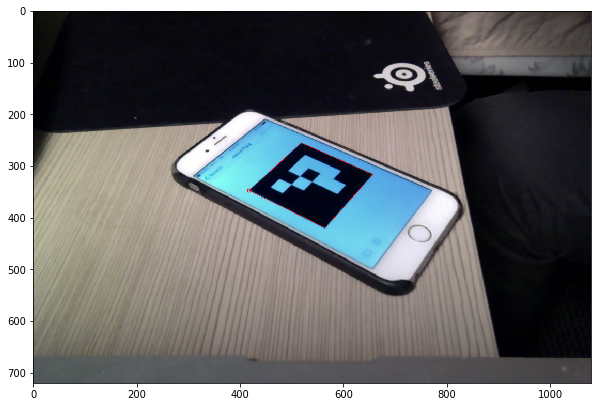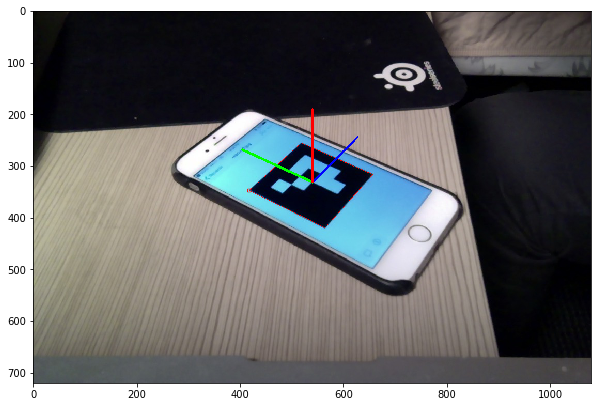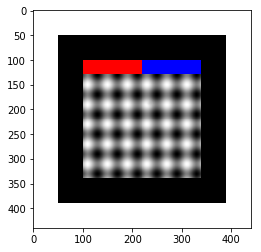# Welcome!!¶

Hello potential employers,

The following is a breif introduction to one of my current side projects which I call FFT Pose Tags. The goal of this notebook is to explain how my implementation works.

## Background: how ArUco tags work¶

Feducial pose tags are images that are used to help cameras identify their position and orientation. They are often used in robotics to help identify world location of a moving robot, and in augmented relity applications to project images onto a object. A popular library for producing and finding these tags is ArUco. Functions for identifying and finding the poses are integrated in the popular OpenCV library.

### This is an image of an ArUco Tag¶

In :
from imageio import imread
import matplotlib.pyplot as plt

plt.figure(figsize=(10,10))
plt.imshow(aruco_tag_img)

Out:
<matplotlib.image.AxesImage at 0x108bad470>

### Identify the marker corners¶

ArUco finds potential marker by looking for quadrilaterals, verifies the marker using the internal pattern, and identify each of the corner locations.

In :
from cv2 import aruco
#the aruco dictionary defines
ARUCO_DICT = aruco.getPredefinedDictionary(aruco.DICT_4X4_50)
(aruco_marker_corners,_,_) = aruco.detectMarkers(aruco_tag_img, ARUCO_DICT)
marked_aruco_img = aruco.drawDetectedMarkers(aruco_tag_img, aruco_marker_corners, borderColor = (255,0,0))

plt.figure(figsize=(10,10))
plt.imshow(marked_aruco_img)

Out:
<matplotlib.image.AxesImage at 0x10559c9e8>### Identify the pose¶

It then uses PnP to caculate the pose

In :
import numpy as np
import pickle
#load the camera parameters which define how pixels translate into directions out of the camera
aruco_pose = aruco.estimatePoseSingleMarkers(corners = aruco_marker_corners,
markerLength = 2,
cameraMatrix = CAMERA_PARAMS['mtx'],
distCoeffs = CAMERA_PARAMS['dist'])

aruco_pose_img = aruco.drawAxis(image = aruco_tag_img,
cameraMatrix = CAMERA_PARAMS['mtx'],
distCoeffs = CAMERA_PARAMS['dist'],
rvec = aruco_pose,
tvec = aruco_pose,
length = 2.);
plt.figure(figsize=(10,10))
plt.imshow(aruco_pose_img)

Out:
<matplotlib.image.AxesImage at 0x105e79e10>## My FFT Pose Tags¶

I have designed my own tags that attempt to alleviate some of the shortcomeings of ArUco and other tags. The idea is to track the changes in frequency that occur as you distort an image by applying affine transformations. This allows us to use the entire image to track the pose which will hopefully help the tags become robust to occlusion, and let us leverage some Fourie transform tecniques to identify the pose with high accuracy.

### My Tag structure:¶

My tag has a few features. The black square boarder is designed so that we can use existing libraries to identify potential locations tags. The blue and red tags on the side are used to easily identify if a potential quadrilateral is a tag. The largest area in the center is a sinusoidal signal extending vertically combined with a sinusoid extending horizontally. This section will be used to decompose the orientation based on how the frequency changes as we rotate the tag.

In :
fft_tag = imread('fft_tag.png')
plt.imshow(fft_tag)

Out:
<matplotlib.image.AxesImage at 0x106f4d400>### Fourier transforms¶

Fourier transforms convert a signal consisting of sinusoids into their a frequency domain. Below you can see the undistorted tag's 2D discreet Fourier transform (an fast implementation an algorithm for solving this iis called FFT). Notice that there are two points on the resulting frequency domain. The points direction should be interpreted as the direction the frequency is extending in, and its magnitude is frequency of that signal.

In :
#crop out the boarder
freq_section = fft_tag[130:340,130:340,:]
freq_section = np.sum(freq_section, axis = 2)
freq_section = freq_section - np.mean(freq_section)

frequencies = np.fft.fftshift(np.fft.fft2(freq_section))

#remove the 0 frequency section
(rows, cols) = frequencies.shape
frequencies[rows//2,cols//2] = 0

#zoom in
frequencies = frequencies[90:-90, 90:-90]

plt.figure(figsize=(10,10))
plt.subplot(121); plt.title("Tag"); plt.imshow(freq_section)
plt.subplot(122); plt.title("Frequencies"); plt.imshow(abs(frequencies.real))

Out:
<matplotlib.image.AxesImage at 0x110799438>